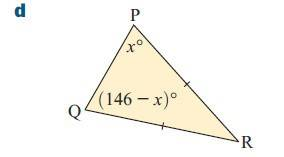# Isosceles triangle angles

## Homework Statement

Find x[/B]a+b+c=180
[/B]

## The Attempt at a Solution

So RP=RQ, the base angles are x and (146-x), normally the base angles are the same but in this scenario they are different.
x+146-x+?=180

## Answers and Replies

DrClaude
Mentor
normally the base angles are the same but in this scenario they are different.
How can you build a isosceles triangle with unequal base angles???

Chestermiller
Mentor
?=34

Ray Vickson
Science Advisor
Homework Helper
Dearly Missed

## Homework Statement

Find x[/B]
View attachment 104589

a+b+c=180
[/B]

## The Attempt at a Solution

So RP=RQ, the base angles are x and (146-x), normally the base angles are the same but in this scenario they are different.
x+146-x+?=180

Why do you say that x and 146-x are different? Wouldn't that depend on the value of x?

I marked this solved

SammyS
Staff Emeritus
Science Advisor
Homework Helper
Gold Member
I marked this solved
What's your solution ?

What's your solution ?
x+146-x+x=180
x+146=180
x=34,
180-34=146
146/2=73
x=73

Last edited:
Chestermiller
Mentor
x+146-x+x=180
x+146=180
x=73
Really? Check your algebra. Also, your equation is incorrect.